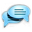## How to locate A Ranger portal with just two flags

. . . and some math.

landmarks == flags

How to locate A Ranger portal with just two measurements

You thought that math in elementary school will never come handy?
Here it is!

You know there is a portal out there.
You know that the Ranger map with the amber can tell you within 500 meters where the portal is.
What it cannot tell you is what direction.
I am going to show you how to figure this out with just two flags.

You will need a piece of paper and pencil, and an arc-cosine table. Alternatively, you can use a spreadsheet like Microsoft Excel or LibreCalc;-)

If we know the length of the three sides of a triangle, any shaped triangle, we can tell each and every angle of it using arc-cosine.

The equation is as follows for each angle:
Let's make the length of each side named a, b, and c, while name the angles A, B, and C.

A=arccos(b^2+c^2-a^2 / 2bc)
B=arccos(a^2+c^2-b^2 / 2ac)
B=arccos(a^2+b^2-c^2 / 2ab)

Let's put this into use to find the portal.

Pull out your map, and let the amber search for the portal.
Your first result is say 78. That means the portal is 78 meters away in any direction.
Drop a flag and name the flag '78'.

Now start out and travel towards any direction.
Pull out your map, and let the amber search for the portal.
The second result is 113. The portal now is 113 meters away, you went the wrong direction.
Drop a flag and name it '113'. Do not move! If you moved off of the flag 113, move back to it until it is saying you are 0 meters away.

Target the flag 78. How far is it? In our example, it is 169 meters away.

Suddenly, now we have the three sides of the triangle.
a=78
b=113
c=169

Do not move! On your compass, right-click and select North. turn until you are facing North, as on the example. The white compass triangle points to the top.

Now, use your elementary school calculation and get the angle for angle B, or the point that is connecting side b to side c.

Put the numbers in your spreadsheet in cells A1, A2, and A3, respectively.

In Excel/LibreCalc/OpenCalc the equation is:
In C1 put the following equation. This calculates the angle for angle B, which is at the point where sides a and side c meet.

=DEGREES(ACOS((A2^2+A3^2-A1^2)/(2*A2*A3)))

My result for this angle is 33.92510046.... Which means nothing unless you have a protractor.

Take a look at 34 degrees. It appears twice on the protractor, on the left of 90 and right of the 90 degrees.
Since the other point is to the left of us, look at the left side.
From look at North (90 degrees), the portal is 34 degrees, and 113 meters away.
If we were at the left flag (flag 78), the calculation would be :

=DEGREES(ACOS((sidea^2+sideb^2-sidec^2)/(2*sidea*sideb)))

My result for that is 123.4160631. Since the other flag is to the right, the portal is 123 degrees, and 78 meters away.

To make it into step actions:

0. get closer than 500 meters to portal
1. measure first distance sidea to portal
2. create flag at current location. Name it the distance to the portal
3. run somewhere else
4. measure second distance sideb to portal
5. measure distance to first, sidea flag, this is sidec
6. look North
6. calculate angle from current spot using sidea, sideb and sidec measurements
=DEGREES(ACOS((sidea^2+sideb^2-sidec^2)/(2*sidea*sideb)))
7. turn to calculated angle and run sideb meters